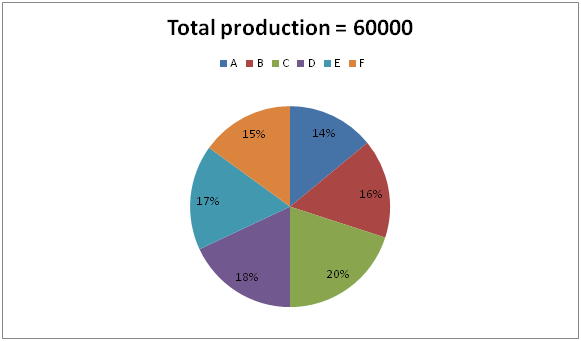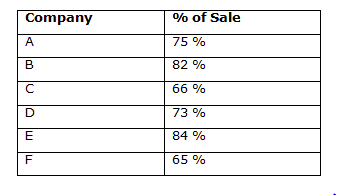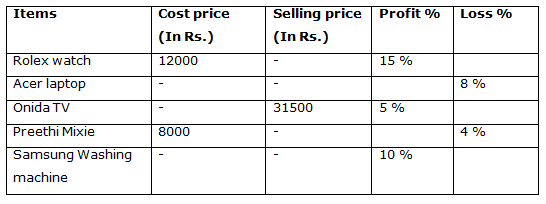# Quantitative Aptitude Questions (Data Interpretation) for NIACL Assistant Mains 2018 Day-203

Dear Readers, NIACL is conducting Online Examination for the recruitment of Assistant. To enrich your preparation here we have providing new series of Data Interpretation – Quantitative Aptitude Questions. Candidates those who are appearing in NIACL Assistant Mains Exams can practice these Quantitative Aptitude average questions daily and make your preparation effective.

Click “Start Quiz” to attend these Questions and view Solutions

Directions (Q. 1 – 5) Study the following information carefully and answer the given questions:

The following pie chart shows the percentage distribution of production of a certain item and the table shows the percentage of sale of different companies in the year 20171) Find the difference between the total sale of company A and C together to that of total sale of company B and E together?

a) 1280

b) 1750

c) 2220

d) 2460

e) None of these

2) Find the ratio between the total production of company B and C together to that of company E and F together?

a) 9: 8

b) 7: 5

c) 3: 1

d) 12: 7

e) None of these

3) Find the average sale of company D, E and F together?

a) 8152

b) 6788

c) 5276

d) 7434

e) None of these

4) Total production of company B is approximately what percentage of total sale of company E?

a) 125 %

b) 112 %

c) 136 %

d) 148 %

e) 95 %

5) Total sale of company C is approximately what percentage more/less than the total production of company A?

a) 6 % less

b) 15 % more

c) 20 % more

d) 6 % more

e) 15 % less

Directions (Q. 6 – 10) Study the following information carefully and answer the given questions:

The following table shows the Cost price, Selling price, Profit % and Loss % of different items in a certain dealer. Some data’s are missing.6) If the ratio between the cost price of Acer laptop and Onida TV is 7: 6, then find the difference between the selling price of Acer laptop to that of Preethi Mixie?

a) Rs. 22350

b) Rs. 18680

c) Rs. 27890

d) 24520

e) None of these

7) If the cost price of Samsung washing machine is 3 times the cost price of Preethi Mixie and discount % is 12 %, then find the marked price for Samsung washing machine?

a) Rs. 28000

b) 30000

c) Rs. 32000

d) Rs. 35000

e) None of these

8) Find the ratio of the cost price of Rolex watch to that of Onida TV?

a) 7: 9

b) 11: 17

c) 2: 5

d) 23: 25

e) None of these

9) Find the total cost price of Rolex watch, Onida TV and Preethi Mixie?

a) 50000

b) Rs. 55000

c) Rs. 45000

d) Rs. 40000

e) None of these

10) The selling price of Acer laptop is approximately what percentage of the selling price of Samsung washing machine, if the cost price of Acer laptop is Rs. 35000 and the cost price of Samsung washing machine is Rs, 24000?

a) 143 %

b) 154 %

c) 166 %

d) 122 %

e) 108 %

Direction (1-5) :

Explanation:

The total sale of company A and C together

= > 60000*(14/100)*(75/100) + 60000*(20/100)*(66/100)

= > 6300 + 7920 = 14220

The total sale of company B and E together

= > 60000*(16/100)*(82/100) + 60000*(17/100)*(84/100)

= > 7872 + 8568 = 16440

Required difference = 16440 – 14220 = 2220

Explanation:

The total production of company B and C together

= > 60000*(36/100)

The total production of company E and F together

= > 60000*(32/100)

Required ratio = [60000*(36/100)] : [60000*(32/100)] = 36 : 32

= > 9 : 8

Explanation:

The total sale of company D, E and F together

= > 60000*(18/100)*(73/100) + 60000*(17/100)*(84/100) + 60000*(15/100)*(65/100)

= > 7884 + 8568 + 5850 = 22302

Required average = 22302/3 = 7434

Explanation:

Total production of company B

= > 60000*(16/100) = 9600

Total sale of company E

= > 60000*(17/100)*(84/100) = 8568

Required % = (9600/8568)*100 = 112 %

Explanation:

Total sale of company C

= > 60000*(20/100)*(66/100) = 7920

Total production of company A

= > 60000*(14/100) = 8400

Required % = [(8400 – 7920)/8400]*100 = 6 % less

Direction (6-10) :

Explanation:

The ratio between the cost price of Acer laptop and Onida TV = 7: 6

The cost price of Onida TV = 31500*(100/105) = Rs. 30000

6’s = 30000

1’s = 5000

The cost price of Acer laptop = 7’s = Rs. 35000

The selling price of Acer laptop = 35000*(92/100) = Rs. 32200

The selling price of Preethi Mixie = 8000*(96/100) = Rs. 7680

The difference between the selling price of Acer laptop to that of Preethi Mixie

= > 32200 – 7680 = Rs. 24520

Explanation:

The cost price of Samsung washing machine

= > 3*the cost price of Preethi Mixie

= > 3*8000 = Rs. 24000

Discount % = 12 %

The selling price of Samsung washing machine

= > 24000*(110/100) = Rs. 26400

The Marked price of Samsung washing machine

= > 26400*(100/88) = Rs. 30000

Explanation:

The cost price of Rolex watch = Rs. 12000

The cost price of Onida TV = 31500*(100/105) = Rs. 30000

Required ratio = 12000: 30000 = 2: 5

Explanation:

The total cost price of Rolex watch, Onida TV and Preethi Mixie

= > 12000 + 31500*(100/105) + 8000

= > 12000 + 30000 + 8000 = Rs. 50000

Explanation:

The selling price of Acer laptop

= > 35000*(92/100) = Rs. 32200

The selling price of Samsung washing machine

= > 24000 * 110/100 = Rs. 26400

Required % = (32200/26400)*100 = 122 %

Daily Practice Test Schedule | Good Luck

 Topic Daily Publishing Time Daily News Papers & Editorials 8.00 AM Current Affairs Quiz 9.00 AM Quantitative Aptitude “20-20” 11.00 AM Vocabulary (Based on The Hindu) 12.00 PM General Awareness “20-20” 1.00 PM English Language “20-20” 2.00 PM Reasoning Puzzles & Seating 4.00 PM Daily Current Affairs Updates 5.00 PM Data Interpretation / Application Sums (Topic Wise) 6.00 PM Reasoning Ability “20-20” 7.00 PM English Language (New Pattern Questions) 8.00 PM